# The advanced Filter ribbon in Excel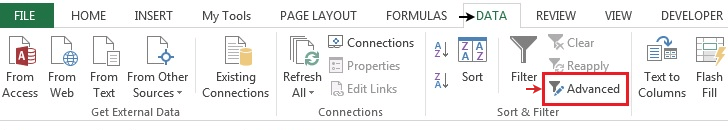Sort and Filter in Excel

Filter option in excel hides the unwanted rows and shows only filtered rows. Rows are filtered by using criteria. Problems occur when you need to filter data many times a day. Either you can apply filters on data and filter each column one by one. This is hectic. Or you can make a sample criteria table and filter data as many times as you like. There are two options to filter. Both ways are mentioned below.

1. Sort & Filter

Sort & Filter option in Excel

Sort And Filter option is usual practice and it's easy to use.

First important step is Select column label of the data. Then Go to Home > Sort & filter > Filter or use keyboard shortcut Ctrl + Shift + L

Advanced sort and filter in Excel

The advanced filtering option in Excel allows you to quickly copy unique information from one table to another. Filtering is a simple, amazing & powerful way to analyze data. Advanced filters are quite easy to use. It allows the user to quickly remove duplicates, extract records that meet certain criteria. It works great when we use wildcards, within 2 date criteria. Here's how you can use Excel's advanced filtering capabilities.

Just Go to Data > Advanced > Select Table range > Select Criteria Range > Ok

Example :

All of these might be confusing to understand. Let's understand how to use the function using an example. Here we have sample customer names having sales, quantity and profit data and we need to apply some filters over the table.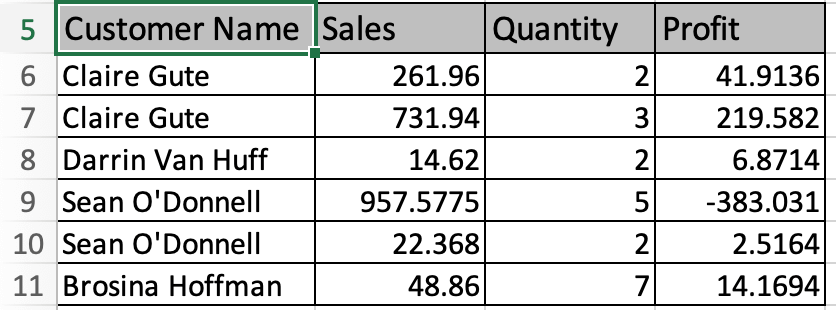We can achieve this task in excel using two ways. First way is using the Standard Filter option.

For this Select any cell with column name(A5:D5). In the above image A5 is selected. Now Either you can Go to Home > Sort & filter > Filter or use keyboard shortcut Ctrl + Shift + L. Your table will be like as shown below.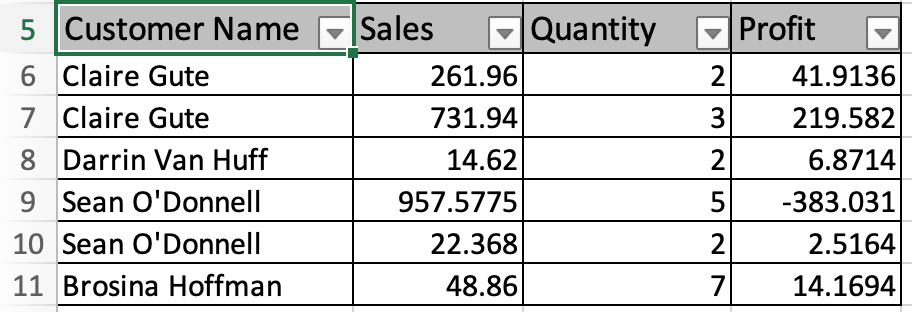Now click the arrow buttons on the columns and Fill the criteria and Click Ok. Now repeat this step on each column where filtering is required. Here filter is applied Sales more 200 & Quantity above 2 & Profit greater than 0.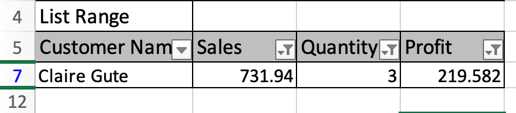Only one row passed through all criterias as shown in the image above.

Alternate method

Another way to go is Advance practice. Create a new table having the same columns and criterias mentioned as shown below.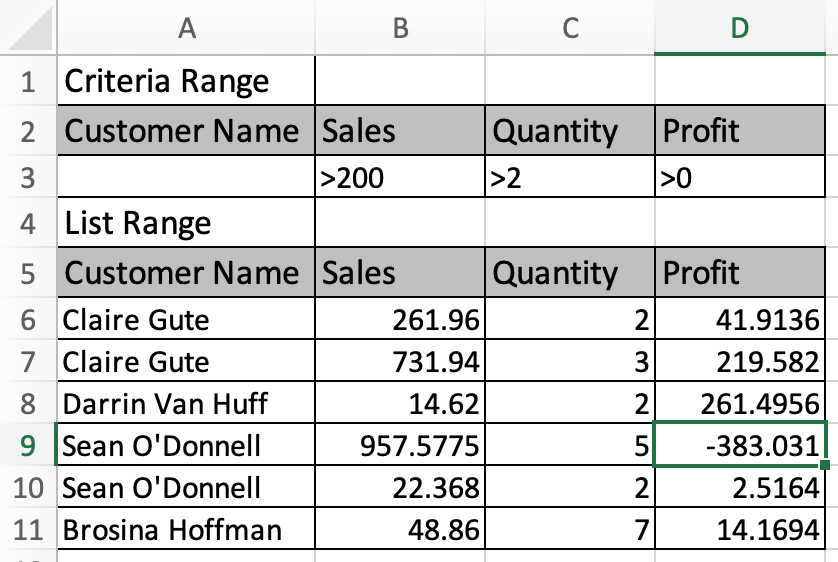Criteria range table is A2:D3 and List range is our data from A5:D11. Now Go to Data > Advanced as shown belowAdvanced filter dialog box appears in front as shown below.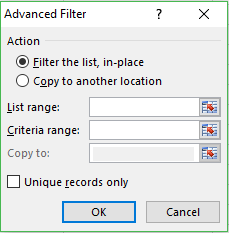Select the List range A5:D11 and Criteria range as A2:D3. Click Ok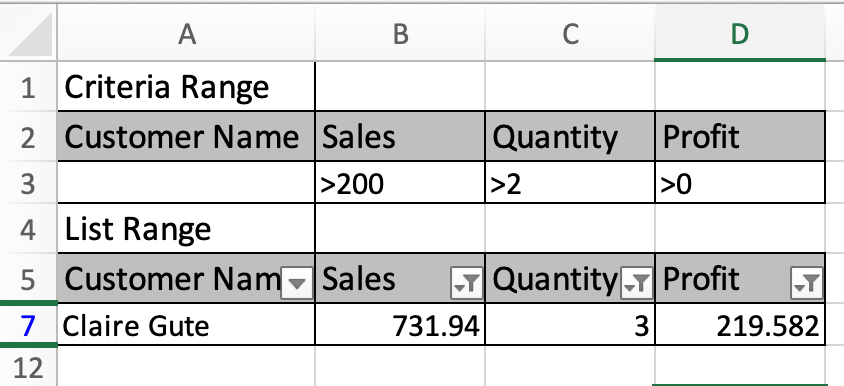Here are all the observational notes using the formula in Excel
Notes :

1. Excel considers a table till a blank row in it. The table below the blank row will be considered the second table. So There must not be a blank row in data or else the sort and filter will be applied only till the last row before the first blank row.
2. Select the top row of the table (column name row) before applying the standard filter option. There is no need to select any cell before proceeding to the advanced filter option.
3. You can add multiple rows in the criteria range to add more criteria under one column like sales between 200 & 300. Date values between two dates.
4. Use wildcards on text columns.

Hope this article about The advanced Filter ribbon in Excel is explanatory. Find more articles on sorting and filtering excel values and related formulas here. If you liked our blogs, share it with your friends on Facebook. And also you can follow us on Twitter and Facebook. We would love to hear from you, do let us know how we can improve, complement or innovate our work and make it better for you. Write to us at info@exceltip.com.

Related Articles :

The SORT Function in Excel 365 (new version) : The SORT function returns the sorted array by the given column number in the array. It also works on horizontal data.

Sort numbers using Excel SMALL Function : To sort numbers using formula in Excel 2016 and older, you can use the SMALL function with other helping functions.

Sort Numeric Values with Excel RANK Function : To sort the numeric values we can use the Rank function. The formula is

Excel Formula to Sort Text : To sort text values using formula in excel we simply use the COUNTIF function. Here is the formula

How To Count Unique Values in Excel With Criteria | To count unique values in excel with criteria we use a combination of functions. The FREQUENCY function is core of this formula

How to use the SUBTOTAL function in Excel: Returns the SUM, COUNT, AVERAGE, STDEV or PRODUCT on applied filtered data in Excel.

Popular Articles :